# Statistics final seminar

5 de Dec de 2016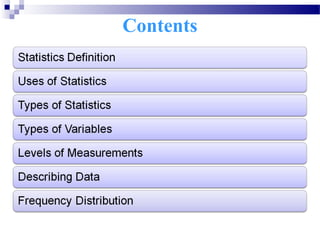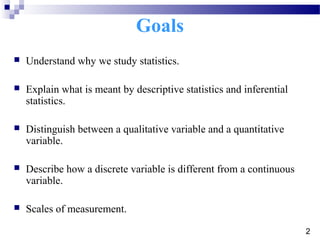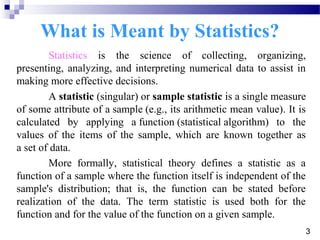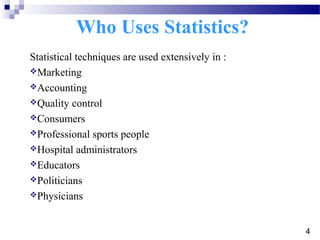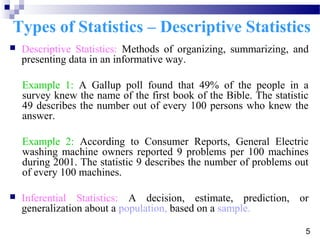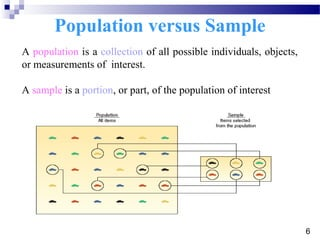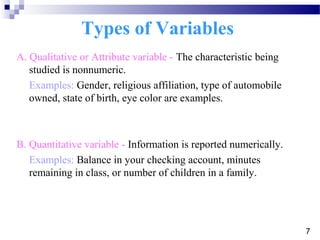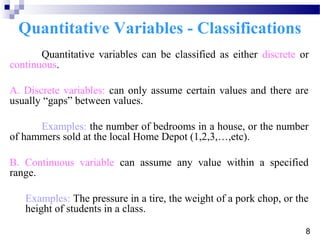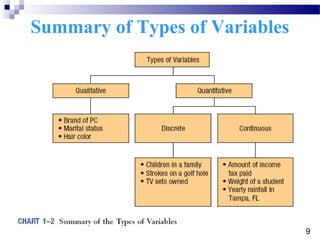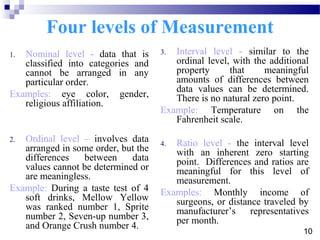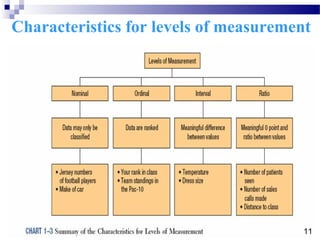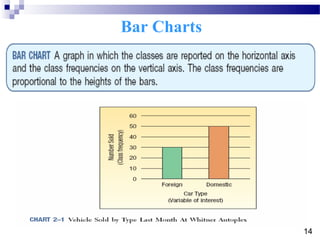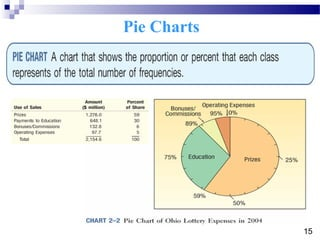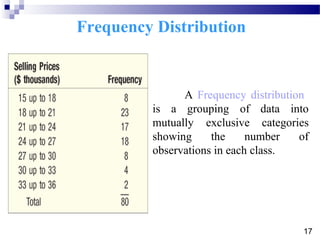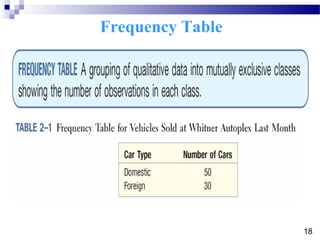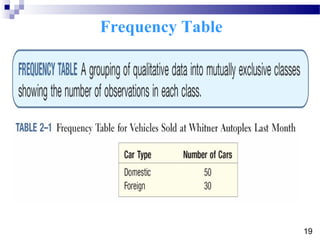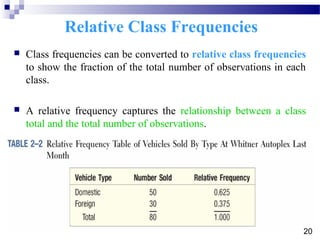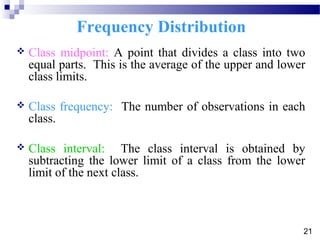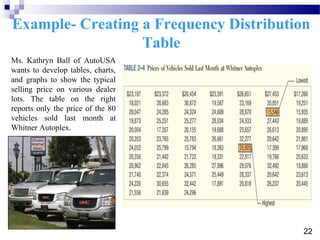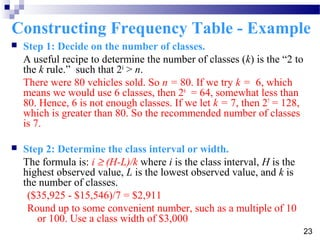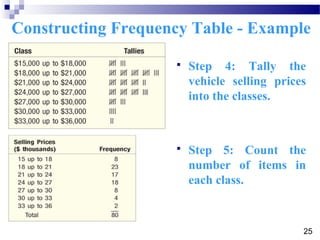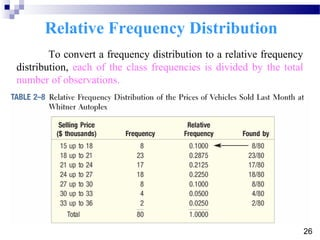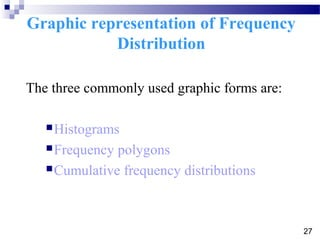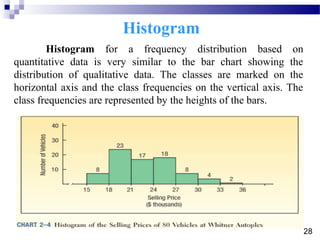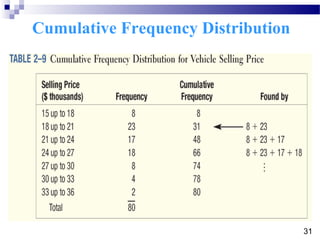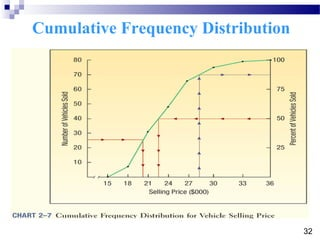1 de 34

### Statistics final seminar

• 1. Contents
• 2. What is Statistics 1
• 3. Goals  Understand why we study statistics.  Explain what is meant by descriptive statistics and inferential statistics.  Distinguish between a qualitative variable and a quantitative variable.  Describe how a discrete variable is different from a continuous variable.  Scales of measurement. 2
• 4. What is Meant by Statistics? Statistics is the science of collecting, organizing, presenting, analyzing, and interpreting numerical data to assist in making more effective decisions. A statistic (singular) or sample statistic is a single measure of some attribute of a sample (e.g., its arithmetic mean value). It is calculated by applying a function (statistical algorithm) to the values of the items of the sample, which are known together as a set of data. More formally, statistical theory defines a statistic as a function of a sample where the function itself is independent of the sample's distribution; that is, the function can be stated before realization of the data. The term statistic is used both for the function and for the value of the function on a given sample. 3
• 5. Who Uses Statistics? Statistical techniques are used extensively in : Marketing Accounting Quality control Consumers Professional sports people Hospital administrators Educators Politicians Physicians 4
• 6. Types of Statistics – Descriptive Statistics  Descriptive Statistics: Methods of organizing, summarizing, and presenting data in an informative way. Example 1: A Gallup poll found that 49% of the people in a survey knew the name of the first book of the Bible. The statistic 49 describes the number out of every 100 persons who knew the answer. Example 2: According to Consumer Reports, General Electric washing machine owners reported 9 problems per 100 machines during 2001. The statistic 9 describes the number of problems out of every 100 machines.  Inferential Statistics: A decision, estimate, prediction, or generalization about a population, based on a sample. 5
• 7. Population versus Sample A population is a collection of all possible individuals, objects, or measurements of interest. A sample is a portion, or part, of the population of interest 6
• 8. Types of Variables A. Qualitative or Attribute variable - The characteristic being studied is nonnumeric. Examples: Gender, religious affiliation, type of automobile owned, state of birth, eye color are examples. B. Quantitative variable - Information is reported numerically. Examples: Balance in your checking account, minutes remaining in class, or number of children in a family. 7
• 9. Quantitative Variables - Classifications Quantitative variables can be classified as either discrete or continuous. A. Discrete variables: can only assume certain values and there are usually “gaps” between values. Examples: the number of bedrooms in a house, or the number of hammers sold at the local Home Depot (1,2,3,…,etc). B. Continuous variable can assume any value within a specified range. Examples: The pressure in a tire, the weight of a pork chop, or the height of students in a class. 8
• 10. Summary of Types of Variables 9
• 11. 1. Nominal level - data that is classified into categories and cannot be arranged in any particular order. Examples: eye color, gender, religious affiliation. 2. Ordinal level – involves data arranged in some order, but the differences between data values cannot be determined or are meaningless. Example: During a taste test of 4 soft drinks, Mellow Yellow was ranked number 1, Sprite number 2, Seven-up number 3, and Orange Crush number 4. 3. Interval level - similar to the ordinal level, with the additional property that meaningful amounts of differences between data values can be determined. There is no natural zero point. Example: Temperature on the Fahrenheit scale. 4. Ratio level - the interval level with an inherent zero starting point. Differences and ratios are meaningful for this level of measurement. Examples: Monthly income of surgeons, or distance traveled by manufacturer’s representatives per month. Four levels of Measurement 10
• 12. Characteristics for levels of measurement 11
• 13. Describing Data: Frequency Tables, Frequency Distributions, and Graphic Presentation 12
• 14. Organize qualitative data into a frequency table. Present a frequency table as a bar chart or a pie chart. Organize quantitative data into a frequency distribution. Present a frequency distribution for quantitative data using histograms, frequency polygons, and cumulative frequency polygons. Goals 13
• 15. Bar Charts 14
• 16. Pie Charts 15
• 17. Pie Chart using Excel 16
• 18. A Frequency distribution is a grouping of data into mutually exclusive categories showing the number of observations in each class. Frequency Distribution 17
• 19. Frequency Table 18
• 20. Frequency Table 19
• 21.  Class frequencies can be converted to relative class frequencies to show the fraction of the total number of observations in each class.  A relative frequency captures the relationship between a class total and the total number of observations. Relative Class Frequencies 20
• 22.  Class midpoint: A point that divides a class into two equal parts. This is the average of the upper and lower class limits.  Class frequency: The number of observations in each class.  Class interval: The class interval is obtained by subtracting the lower limit of a class from the lower limit of the next class. Frequency Distribution 21
• 23. Ms. Kathryn Ball of AutoUSA wants to develop tables, charts, and graphs to show the typical selling price on various dealer lots. The table on the right reports only the price of the 80 vehicles sold last month at Whitner Autoplex. Example- Creating a Frequency Distribution Table 22
• 24.  Step 1: Decide on the number of classes. A useful recipe to determine the number of classes (k) is the “2 to the k rule.” such that 2k > n. There were 80 vehicles sold. So n = 80. If we try k = 6, which means we would use 6 classes, then 26 = 64, somewhat less than 80. Hence, 6 is not enough classes. If we let k = 7, then 27 = 128, which is greater than 80. So the recommended number of classes is 7.  Step 2: Determine the class interval or width. The formula is: i ≥ (H-L)/k where i is the class interval, H is the highest observed value, L is the lowest observed value, and k is the number of classes. (\$35,925 - \$15,546)/7 = \$2,911 Round up to some convenient number, such as a multiple of 10 or 100. Use a class width of \$3,000 Constructing Frequency Table - Example 23
• 25.  Step 3: Set the individual class limits Constructing Frequency Table - Example 24
• 26.  Step 4: Tally the vehicle selling prices into the classes.  Step 5: Count the number of items in each class. Constructing Frequency Table - Example 25
• 27. To convert a frequency distribution to a relative frequency distribution, each of the class frequencies is divided by the total number of observations. Relative Frequency Distribution 26
• 28. The three commonly used graphic forms are: Histograms Frequency polygons Cumulative frequency distributions Graphic representation of Frequency Distribution 27
• 29. Histogram for a frequency distribution based on quantitative data is very similar to the bar chart showing the distribution of qualitative data. The classes are marked on the horizontal axis and the class frequencies on the vertical axis. The class frequencies are represented by the heights of the bars. Histogram 28
• 30. Histogram Using Excel 29
• 31.  A frequency polygon also shows the shape of a distribution and is similar to a histogram.  It consists of line segments connecting the points formed by the intersections of the class midpoints and the class frequencies. Frequency Polygon 30
• 32. Cumulative Frequency Distribution 31
• 33. Cumulative Frequency Distribution 32